Question-and-Answer Resource for the Building Energy Modeling Community
Get started with the Help page

# How does EnergyPlus calculate floor U-values?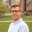I have been looking at the Envelope Summary in the Energy Plus Results for some of my models of domestic buildings and found that the ground floor u-value is very high.

The construction is a simple 10mm of Ceramic Tile and 150mm of Concrete and I would have expected it to be far lower than this. How does OS calculate the U-value? Does OS take into account the exposed perimeter of the floor when calculating this? If so, is there a way of setting the exposed perimeter as my models have party walls with buildings that are not drawn in the model.

Thanks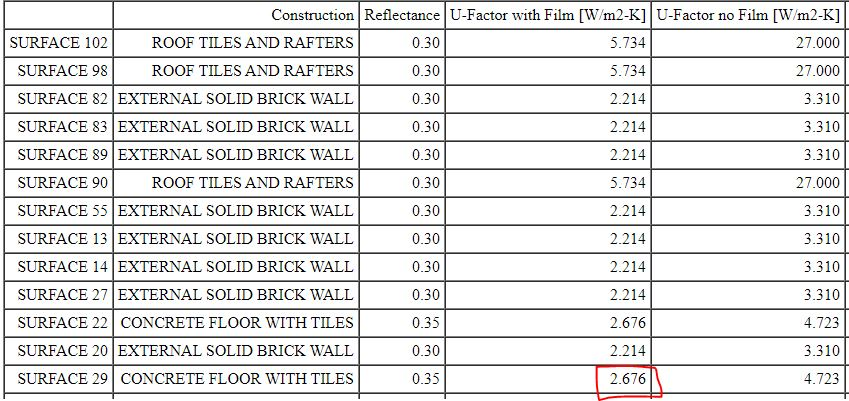Surface 29 is a simple solid floor made of: 10mm of ceramic floor tile with a thermal conductivity of 0.8 W/mK 150mm of concrete with a thermal conductivity of 1.7296 W/mK

I have calculated the solid floor U-value using the CIBSE Guide A method and found the U-value to be 1.625 W/m2K. Please see the methods and calculations below.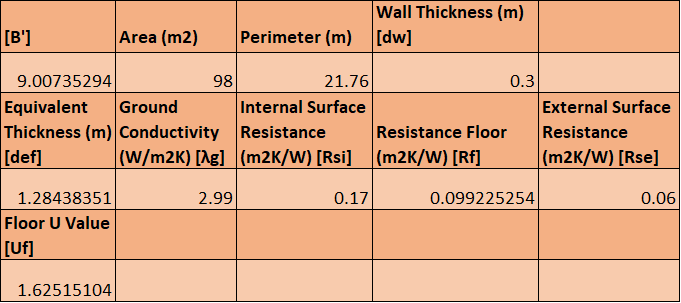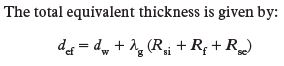(CIBSE Guide A)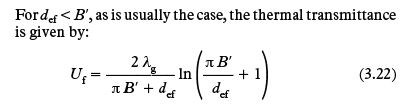(CIBSE Guide A)

edit retag close merge delete

This question is really about EnergyPlus, not OpenStudio, so I'm going to rename/retag it.

I still have not been able to find out how EnergyPlus calculates this floor u-value, I am thinking that maybe I should try to amend the construction to reflect my own calculations using a no mass material on top of the existing structure? Does this sound like something that anyone has done before?

Can you either provide a link to your IDF or share the relevant construction and materials for Surface 29?

Hi @shorowit, yes of course! Surface 29 is a simple solid floor made of: 10mm of ceramic floor tile with a thermal conductivity of 0.8 W/mK 150mm of concrete with a thermal conductivity of 1.7296 W/mK

I have calculated the thermal transmittance of a solid floor using CIBSE Guide A and found the u-value to be 1.625 W/m2K. I will edit the question to show how I calculated this. I am just intrigued to see how EnergyPlus got such a different answer and also I have not set the exposed perimeter in my model, so do not know how it should know this?

Sort by » oldest newest most voted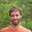It sounds like you're conflating the construction assembly U-factor with the actual ground heat transfer model. The Envelope Summary simply reports construction assembly U-factors, whether floor or any other surface, which is the U-factor through all of the constructions' materials (plus interior/exterior film coefficients as applicable). So it will not take into account soil properties, exposed perimeter, ground temperatures, or anything else like that.

This construction assembly U-factor will then be used by whatever ground heat transfer model you have chosen based on the floor surface's outside boundary condition -- e.g., "Foundation", "Ground", "GroundFCfactorMethod", etc.

If you are trying to verify that the ground heat transfer is appropriate, you might instead look at the EnergyPlus timeseries outputs related to surface heat gain.

more

@shorowit Thank you so much! Yes I was hoping that something similar was the case! Thank you so much for clarifying! After looking at some more posts and the input output reference, it seems that there are several Surface Output Variables for heat transfer, for conduction, radiation, convection. E.g "Surface Outside Face Conduction Heat Transfer Rate per Area [W/m2]". I have downloaded the Add Output Variable measure but just want to find the total heat transfer rate through a surface in Wm2K. Could you advise which Variable to use and how I might be able to apply it in my model?
Thanks!